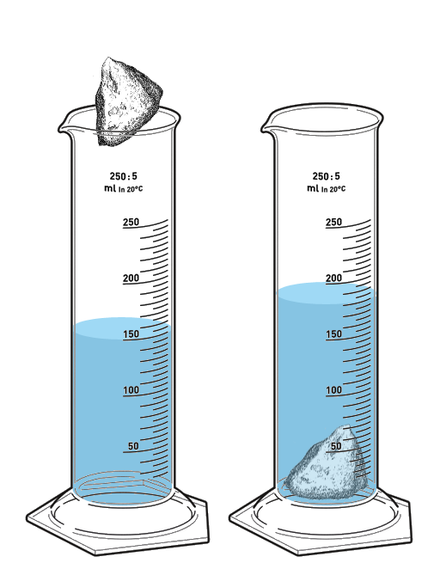Jump to content

# Weight of copper in water

## Recommended Posts

A scale reads 380 N when a piece of copper is hanging from it. What does it read (in N) when it is lowered so that the copper is submerged in water?

I need to find out the mass of the copper right to know how much water it displaced then take the 380 minus the weight of that water?

##### Share on other sites
33 minutes ago, brickman7713 said:
A scale reads 380 N when a piece of copper is hanging from it. What does it read (in N) when it is lowered so that the copper is submerged in water?

I need to find out the mass of the copper right to know how much water it displaced then take the 380 minus the weight of that water?

Yes, you need to find the weight of water which was displaced by the object which was submerged. It will give you the buoyant force expressed in Newtons (weight is always measured in Newtons)

##### Share on other sites

Ok so how would I do that, Do I need to find the size of the object?

##### Share on other sites
21 minutes ago, brickman7713 said:

Ok so how would I do that, Do I need to find the size of the object?

Yes, calculating the volume of the piece of copper is a good start!

##### Share on other sites

Ok but how? I know the mass density is 8,930 kg/m3 and the weight density is 557 lb/ft3 and of course weight of 380 newtons.

##### Share on other sites
12 minutes ago, brickman7713 said:

Ok but how? I know the mass density is 8,930 kg/m3 and the weight density is 557 lb/ft3 and of course weight of 380 newtons﻿.﻿

Let's try this hint, in the form of a question: What mass would make the scale show 380 N?

##### Share on other sites

The figure in pounds and feet isn't likely to help in a science question.

Is there any other data given?
In particular, does it say if this experiment is on the Moon, or Earth or where?

##### Share on other sites
8 minutes ago, John Cuthber said:

The figure in pounds and feet isn't likely to help in a science question.

Is there any other data given?
In particular, does it say if this experiment is on the Moon, or Earth or where?

I assume earth so 9.8 is what I think you are referring to.

9 minutes ago, Ghideon said:

Let's try this hint, in the form of a question: What mass would make the scale show 380 N?

38.75kg ?

##### Share on other sites
21 minutes ago, brickman7713 said:

38.75k﻿g ?

Next hint: what volume of a piece of copper would have that specific mass? Could that volume help you find out how much water that is displaced?
Note; I'm assuming a solid piece of copper, not a hollow sphere or similar.

(You might want to check the rounding of the intermediate steps depending on the precision required in the final answer)

##### Share on other sites
13 minutes ago, Ghideon said:

Next hint: what volume of a piece of copper would have that specific mass? Could that volume help you find out how much water that is displaced?
Note; I'm assuming a solid piece of copper, not a hollow sphere or similar.

(You might want to check the rounding of the intermediate steps depending on the precision required in the final answer)

Is it 45.48 liters in volume then?

##### Share on other sites
8 hours ago, brickman7713 said:

Is it 45.48 liters in volume then?

That number doesn't seem quite right. Compare a volume of 45.48 liters of solid copper with the mass you calculated, what does that show? You might need to show the calculations you have done so far, that would allow for better hints about how to proceed.

##### Share on other sites
9 hours ago, Ghideon said:

That number doesn't seem quite right. Compare a volume of 45.48 liters of solid copper with the mass you calculated, what does that show? You might need to show the calculations you have done so far, that would allow for better hints about how to proceed.

Ok, that was an online tool. How does converting a mass that is solid to a liter that is liquid work?

##### Share on other sites
3 hours ago, brickman7713 said:

How does converting a mass that is solid to a liter that is liquid work?

Mass per unit volume is known as density, often denoted by letter $\rho$. Mass, density and volume is related: $\rho=\frac{m}{V}$. Since copper has $\rho=8930 kg/m^{3}$ that equals $\rho=8.930 kg/dm^{3}$. Note that $1dm^{3}=1Liter$.

So, given the mass of copper you should be able to calculate the volume of copper which equals the volume of water that is displaced. Note that Solid or liquid does not matter, the concept of volume is the same. Here is a picture of measurement of volume by displacement. The amount by which the liquid rises in the cylinder is equal to the volume of the solid rock.Edited by Ghideon

## Create an account or sign in to comment

You need to be a member in order to leave a comment

## Create an account

Sign up for a new account in our community. It's easy!

Register a new account

## Sign in

Already have an account? Sign in here.

Sign In Now
×

• #### Activity

• Leaderboard
×
• Create New...

## Important Information

We have placed cookies on your device to help make this website better. You can adjust your cookie settings, otherwise we'll assume you're okay to continue.